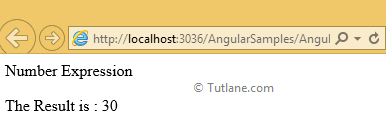# Number Expressions in AngularJS with Examples

Here we will learn what are the number expressions in angularjs and how to use number expressions in angularjs with example.

## AngularJS Number Expressions

As the name itself states that if any expression is using the number and the operators (like , -, *, %, etc) then those are called number expressions. We will see how to use number expression in angularjs with example.

## Example of AngularJS Number Expressions

Create an Empty Web Application in your JavaScript IDE (there are many IDEs available like AptanaStudio, WebStorm etc.) and add an HTML page (say index.html). After adding index.html page add following AngularJs code sample header section of your page

<script type="text/javascript">

var app = angular.module('expressionApp', [])

app.controller("expressionController", function (\$scope) {

\$scope.expression1 = 10;

\$scope.expression2 = 20

});

</script>

Write the following code under the starting of body tag <body> for number expression like as shown below.

<body ng-app="expressionApp">

<form id="form1">

<div ng-controller="expressionController">

<div>Number Expression</div>

<p>The Result is : {{expression1 + expression2}}</p>

</div>

</form>

</body>

Following is the full example code for number expression with live demo as show below

<html xmlns="http://www.w3.org/1999/xhtml">

<title>Number Expression of AngularJs</title>

<script type="text/javascript">

var app = angular.module('expressionApp', [])

app.controller("expressionController", function (\$scope) {

\$scope.expression1 = 10;

\$scope.expression2 = 20

});

</script>

<body ng-app="expressionApp">

<form id="form1">

<div ng-controller="expressionController">

<div>Number Expression</div>

<p>The Result is : {{expression1 + expression2}}</p>

</div>

</form>

</body>

</html>

Now run the number expressions in angularjs example and check output that will be like as shown below

## Output of AngularJS Number Expressions Example

Following is the result of angularjs number expressions example